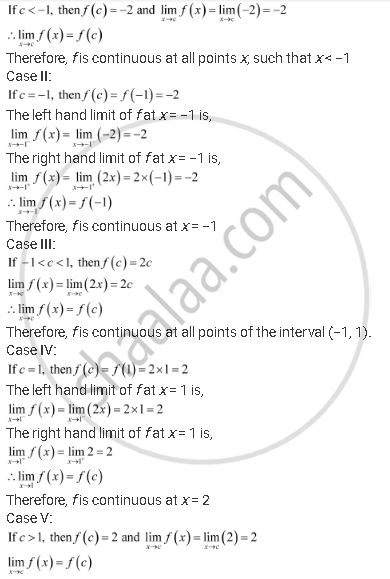Share

# Discuss the Continuity of the Function F, Where F Is Defined by -1, 2x,2 - CBSE (Commerce) Class 12 - Mathematics

#### Question

Discuss the continuity of the function f, where f is defined by

f(x) = {(-2,"," if x <= -1),(2x, "," if -1 < x <= 1),(2, "," if x > 1):}

#### Solution

The given function f is f(x) = {(-2,"," if x <= -1),(2x, "," if -1 < x <= 1),(2, "," if x > 1):}

The given function is defined at all points of the real line.

Let c be a point on the real line.

Case I:Therefore, f is continuous at all points x, such that x > 1

Thus, from the above observations, it can be concluded that f is continuous at all points of the real line.

Is there an error in this question or solution?

#### APPEARS IN

NCERT Solution for Mathematics Textbook for Class 12 (2018 to Current)
Chapter 5: Continuity and Differentiability
Q: 16 | Page no. 160

#### Video TutorialsVIEW ALL 

Solution Discuss the Continuity of the Function F, Where F Is Defined by -1, 2x,2 Concept: Continuous Function of Point.
S